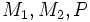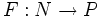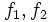Cobordism of smooth maps

(diff) ← Older revision | Latest revision (diff) | Newer revision → (diff)
Suppose$M_1, M_2, P$ are differential manifolds, and$f_1:M_1 \to P, f_2:M_2 \to P$ are smooth maps. Then, a smooth cobordism from$f_1$ to$f_2$ is the following data:
• A smooth cobordism from$M_1$ to$M_2$ i.e. a manifold with boundary$(N, \partial N)$ with a diffeomorphism$M_1 \sqcup M_2 \to \partial N$
• A smooth map$F:N \to P$ such that the composite of$F$ with the inclusions of$M_1, M_2$ are respectively the maps$f_1, f_2$
Note that we may sometimes fix the cobordism between$M_1$ and$M_2$, and ask whether we can fill in a function$F$ for the particular cobordism.